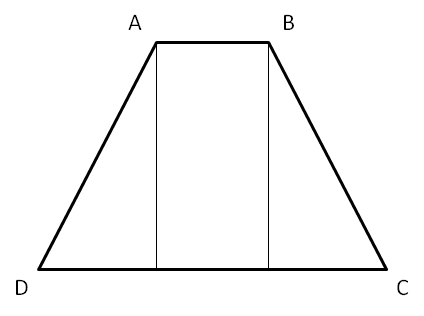Area of trapezium

## Objective:

To show that the area of a trapezium is equal to half the product of its altitude and sum of its parallel sides.

### Theory

1. Trapezium is a quadrilateral with one pair of opposite sides parallel.
2. A quadrilateral is a parallelogram if a pair of its opposite sides are parallel and equal to each other.
3. The parallel sides are called "bases" of the trapezium and the other two sides are called the "legs" of trapezium.
4. Area of parallelogram = base(b) X height(h)

## Proof:

Consider trapezium ABCD. AB and DC are the bases (parallel sides) and h is the height of trapezium ABCD.A parallelogram can be formed by creating a copy of trapezium ABCD and placing it inverted touching side BC as shown in figure below:We observe that parallelogram ASPD is formed by combining two trapeziums ABCD and BSPC.

∴ Area of trapezium ABCD     = 1/2 X Area of parallelogram ASPD
= ½ X DP X h
= 1/2 X (DC+CP) X h
=1/2 X (b1 + b2) X h
= ½ X (AB + DC) X h

Thus, area of a trapezium is equal to half the product of its altitude and sum of its parallel sides.

### Example:-

Find the area of the following trapezium.### Solution:-

Given,

b1= 5 cm
b2= 11 cm
h= 8 cm

Area of trapezium = 1/2(b1+b2) X h

=1/2(11+5) X 8

=64 cm²